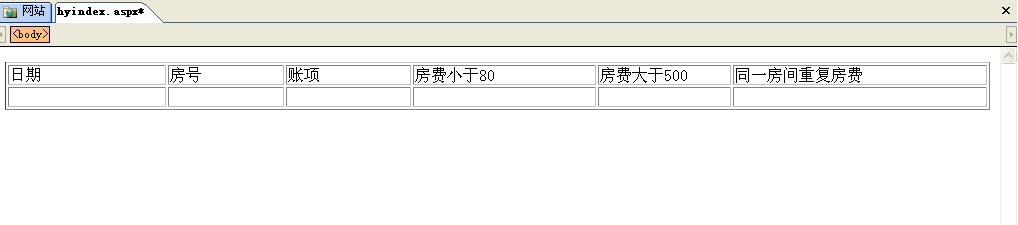WHERE (YEAR([Date]) = YEAR({ fn NOW() })) AND (MONTH([Date]) = MONTH({ fn NOW() }))
AND (DAY([Date]) = DAY({ fn NOW() }))

root字段中的房间号
WHERE (Room > 301) AND (Room < 711)

tanascode字段（小于3的值）
WHERE (TransCode <= 2)

amount字段（中小于1大于500的值）
WHERE (Amount > 0) AND (Amount < 500)

1个回答

`````` 你用ado查询数据库，将结果填入表格：
<table>
<th>
<td>日期</td>
<td>房号</td>
...
</th>
<tr>
<% while not rs.eof %>
<td><% rs!字段1 %></td>
<td><% rs!字段2 %></td>
...
<% wend %>
</tr>
``````xj0999 查询语句不知道在ASP中怎么写，在SQL中查询我会，
5 年多之前 回复xj0999 就是这一步不会啊
5 年多之前 回复